Test: Magnetostatics - 1

# Test: Magnetostatics - 1 - Electrical Engineering (EE)

Test Description

## 10 Questions MCQ Test Topicwise Question Bank for Electrical Engineering - Test: Magnetostatics - 1

Test: Magnetostatics - 1 for Electrical Engineering (EE) 2023 is part of Topicwise Question Bank for Electrical Engineering preparation. The Test: Magnetostatics - 1 questions and answers have been prepared according to the Electrical Engineering (EE) exam syllabus.The Test: Magnetostatics - 1 MCQs are made for Electrical Engineering (EE) 2023 Exam. Find important definitions, questions, notes, meanings, examples, exercises, MCQs and online tests for Test: Magnetostatics - 1 below.
Solutions of Test: Magnetostatics - 1 questions in English are available as part of our Topicwise Question Bank for Electrical Engineering for Electrical Engineering (EE) & Test: Magnetostatics - 1 solutions in Hindi for Topicwise Question Bank for Electrical Engineering course. Download more important topics, notes, lectures and mock test series for Electrical Engineering (EE) Exam by signing up for free. Attempt Test: Magnetostatics - 1 | 10 questions in 30 minutes | Mock test for Electrical Engineering (EE) preparation | Free important questions MCQ to study Topicwise Question Bank for Electrical Engineering for Electrical Engineering (EE) Exam | Download free PDF with solutions
 1 Crore+ students have signed up on EduRev. Have you?
Test: Magnetostatics - 1 - Question 1

### The magnitude of H at a radius of 1 meter from along linear conductor is 1 A/m. The current in the wire is

Detailed Solution for Test: Magnetostatics - 1 - Question 1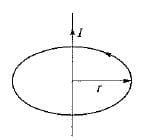Given, r = 1 m, H = 1 A/m
Using Ampere’s circuit law, we have: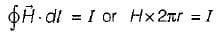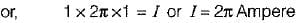Test: Magnetostatics - 1 - Question 2

### What is the value of magnetic flux density at the centre of the square current loop shown in figure below?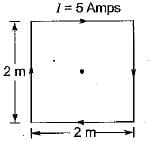Detailed Solution for Test: Magnetostatics - 1 - Question 2

The magnetic flux density at the centre of the square having each side a is given by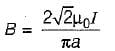Given, a = 2 m, I = 5 Amp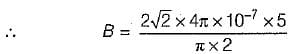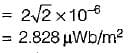Test: Magnetostatics - 1 - Question 3

### A solenoid of 20 cm long and 1 cm diameter has a uniform winding of 1000 turns. If the solenoid is placed in a uniform field of 2 Wb/m2 flux density and a current of 10 amps, is passed through the solenoid winding, then the maximum torque on the solenoid will be

Detailed Solution for Test: Magnetostatics - 1 - Question 3

Given, l = 20 cm = 0.2 m,
r - 0.5 cm = radius = 0.005 m
N = 1000,
B = 2 Wb/m2,
I = 10 A
The torque acting on the solenoid is
T = NIAB sinθ
T will be maximum when
θ = 90°, i.e. sin90° = 1
∴ Tmax = 1000 x 10 (0.005 x 0.2) x 2
= 20 N-m

Test: Magnetostatics - 1 - Question 4

What is the value of magnetic flux density at the centre of a current carrying loop when the loop radius is 2 cm, loop current is 1 mA and the loop is placed in air?

Detailed Solution for Test: Magnetostatics - 1 - Question 4

Magnetic flux density at the centre of a current carrying loop is given by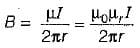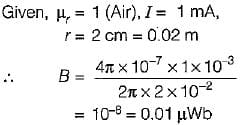Test: Magnetostatics - 1 - Question 5

A magnetic field known to be directed in a cartesian co-ordinate system so that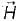exists in the x-direction where Hx = Constant. The value of curl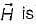Detailed Solution for Test: Magnetostatics - 1 - Question 5

Curl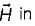cartesian coordinate is given by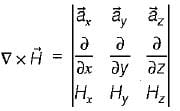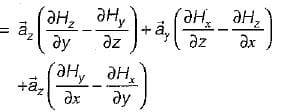Since Hx = constant and Hy = Hz = 0, therefore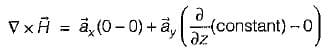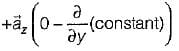= 0 + 0 + 0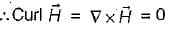Test: Magnetostatics - 1 - Question 6

Match List-I with List-ll and select the correct answer using the codes given below the lists:
List-I
B. Maxwell equation in point form
C. Electric field strength general equation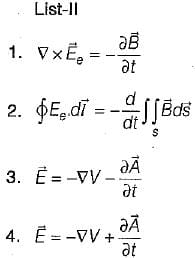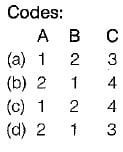Test: Magnetostatics - 1 - Question 7

The force acting between two parallel wires carrying currents I1 and I2 and separated by a distance r is given by

Test: Magnetostatics - 1 - Question 8

Flux lines are received at an iron-air boundary at an angle of 45° from the normal on the iron side of the boundary. If the iron has a relative permeability of 350, then the angle from the normal with which the flux emerges into the air would be

Detailed Solution for Test: Magnetostatics - 1 - Question 8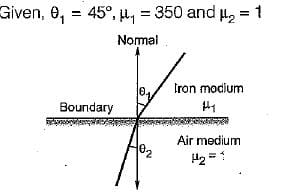Let medium-1 and medium-2 are respectively iron and air, then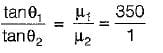or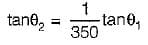or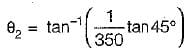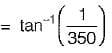Test: Magnetostatics - 1 - Question 9

The unit of magnetic flux is given by

Test: Magnetostatics - 1 - Question 10

Assertion (A): The potential in case of magnetic field is a vector potential.
Reason (R): The potential in case of electric field is a scalar potential

Detailed Solution for Test: Magnetostatics - 1 - Question 10
• Both assertion and reason are correct statements.
• The potential in case of magnetic field is a vector potential because source for producing a magnetic field is a current element which has both magnitude and direction.
• The potential in case of electric field i.e. electric potential is a scalar potential because the source for producing an electric field is a charge which is a scaler quantity (having only magnitude).

## Topicwise Question Bank for Electrical Engineering

211 tests
Information about Test: Magnetostatics - 1 Page
In this test you can find the Exam questions for Test: Magnetostatics - 1 solved & explained in the simplest way possible. Besides giving Questions and answers for Test: Magnetostatics - 1, EduRev gives you an ample number of Online tests for practice

## Topicwise Question Bank for Electrical Engineering

211 tests(Scan QR code)